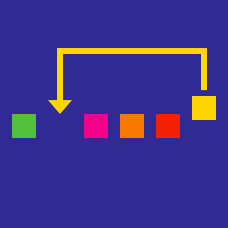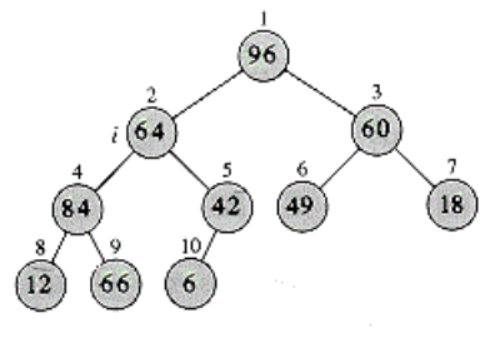Computer Science

# Heap Sort

A crucial element of the Heap_Sort is the construction of the heap itself. This is achieved by calling a heapify procedure on some nodes until all nodes satisfy the Max_Heap property( Any node must have a value less or equal to that of its parent ).

The node in position $2$ violates the heap property. Once heapify is applied to it, which position will it be in?1 2 3 4 5 6 7 def Heap_Sort( A ): Build_Heap(A) for i in range(A.size, 1, -1): #loop down from A.size to 2 # !MISSING LINE !!! A.size -= 1 Max_Heapify(A,1) return list(A.A) 

Consider the Heap_Sort method above. Given a list of numbers A it will construct a heap and sort them. One line is however missing(line 4). What line of code should be there in order to have a properly functioning Heap_Sort algorithm.

Note

• The method assumes that the index of the first item is $1$ not $0$.

A crucial element of the Heap_Sort is the construction of the heap itself. This is achieved by calling a heapify procedure on some nodes until all nodes satisfy the Max_Heap property( Any node must have a value less or equal to that of its parent ).

Suppose we have a an array with $51$ elements. At most, how many calls to heapify are required to convert it to a heap?

×